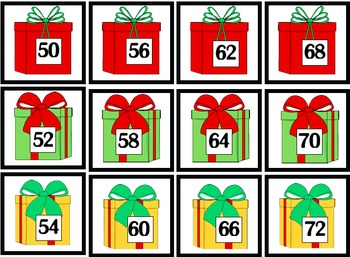# Christmas Math CentersResource Type
Format
PDF (6 MB|55 pages)
\$4.00
\$4.00

### Description

These math centers allow students to work and manipulate numbers to help them truly understand addition concepts. Students will work with numbers on a deeper level as they find missing addends as well as create fact families.

All of these centers also have a worksheet component that allows the teacher to hold students accountable for their center work as well as to check for understanding with each of these activities. There are two versions of each worksheet for differentiated learning.

In these centers students will:
*Help Santa find the missing number in an addition sentence for example 3+__=10.
*Match stockings to find fact families
*Use presents to order numbers from 2-120 (counting by 2s,) 5-120 (counting by 5s,) and 10-120 (counting by 10s.)

All of these centers are aligned with the common core:

CC.K.CC.1 Know number names and the count sequence. Count to 100 by tens.

CC.1.OA.4 Understand and apply properties of operations and the relationship between addition and subtraction. Understand as an unknown-addend problem. For Example, subtract 10-8 by finding the number that makes 10 when added to 8.

CC.1.OA.3 Understand and apply properties of operations and the relationship between addition and subtraction. Apply properties of operations as strategies to add and subtract. Examples: If 8+3=11 is known, then 3+8=11 is also known. (Communicative property of addition.)

CC.1.OA.5 Add and subtract within 20. Relate Counting to addition and subtraction (e.g., by counting on to add 2.)

CC.2.01.2 Add and subtract within 20. Fluently add and subtract within 20 using mental math strategies know from memory all sums of two one-digit numbers.
Total Pages
55 pages
N/A
Teaching Duration
N/A
Report this Resource to TpT
Reported resources will be reviewed by our team. Report this resource to let us know if this resource violates TpT’s content guidelines.